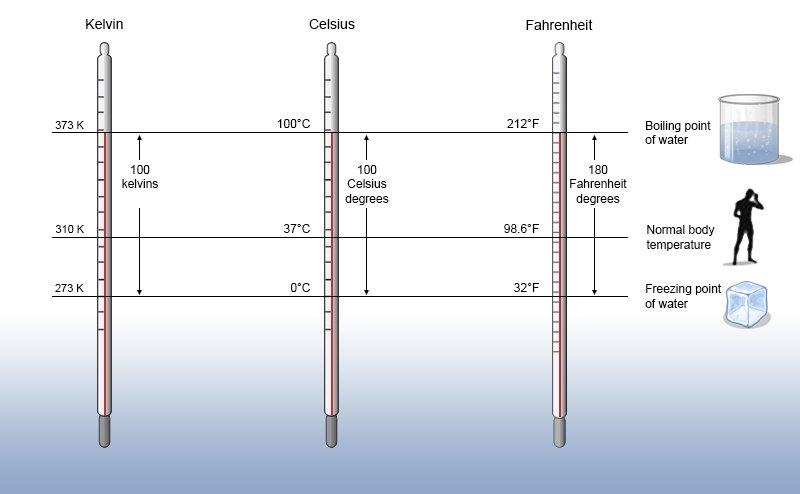# 1.2: The meaning and measurement of temperature

$$\newcommand{\vecs}{\overset { \rightharpoonup} {\mathbf{#1}} }$$ $$\newcommand{\vecd}{\overset{-\!-\!\rightharpoonup}{\vphantom{a}\smash {#1}}}$$$$\newcommand{\id}{\mathrm{id}}$$ $$\newcommand{\Span}{\mathrm{span}}$$ $$\newcommand{\kernel}{\mathrm{null}\,}$$ $$\newcommand{\range}{\mathrm{range}\,}$$ $$\newcommand{\RealPart}{\mathrm{Re}}$$ $$\newcommand{\ImaginaryPart}{\mathrm{Im}}$$ $$\newcommand{\Argument}{\mathrm{Arg}}$$ $$\newcommand{\norm}{\| #1 \|}$$ $$\newcommand{\inner}{\langle #1, #2 \rangle}$$ $$\newcommand{\Span}{\mathrm{span}}$$ $$\newcommand{\id}{\mathrm{id}}$$ $$\newcommand{\Span}{\mathrm{span}}$$ $$\newcommand{\kernel}{\mathrm{null}\,}$$ $$\newcommand{\range}{\mathrm{range}\,}$$ $$\newcommand{\RealPart}{\mathrm{Re}}$$ $$\newcommand{\ImaginaryPart}{\mathrm{Im}}$$ $$\newcommand{\Argument}{\mathrm{Arg}}$$ $$\newcommand{\norm}{\| #1 \|}$$ $$\newcommand{\inner}{\langle #1, #2 \rangle}$$ $$\newcommand{\Span}{\mathrm{span}}$$$$\newcommand{\AA}{\unicode[.8,0]{x212B}}$$

One of the key ways that we will need to describe energy is in direct connection to the temperature of a system. One of the foundational ideas of statistical mechanics is a model for the behavior of gas molecules called the kinetic theory of gases - it’s a model built on considering the particles in a gas to be very small, noninteracting, and colliding in perfectly elastic ways. (The Wikipedia article on the kinetic theory is a wonderful primer with a very understandable derivation.) The kinetic theory also provides our first definition of temperature, which is a measure of the average kinetic energy of the particles of a gas at a state of thermal equilibrium. Mathematically, that measure is

$upper T equals StartFraction 2 upper K Subscript t o t Baseline Over 3 upper N k Subscript upper B Baseline EndFraction Units colon upper K$

We can prove this result with the kinetic theory, but we will take it as a definition for now. The average kinetic energy is hidden in the expression of total kinetic energy divided by N, the count of molecules present.

This forces the units of the genuinely new quantity kB to be Joules per Kelvin. This is a constant, our first of the course; it is known as the Boltzmann constant, after the German thermodynamics scholar Ludwig Boltzmann who first described it:

$k Subscript upper B Baseline equals 1.381 times 10 Superscript negative 23 Baseline upper J upper K Superscript negative 1$

We will explore the meaning of this constant and its connection to other ideas in thermodynamics as we move forward in the course. Multiplying kB by temperature gives units of energy, and it is not uncommon when talking about the energy of individual molecules to describe their energy in units of kBT.

The direct proportionality between average kinetic energy of molecules and temperature also enforces a system of measuring temperature where the temperature is zero when the average kinetic energy of all molecules is zero - a circumstance whose existence is only theoretical, never actually observed. It also enforces the impossibility of negative temperature. We call the Kelvin scale an absolute scale for that reason - every temperature is positive and has a neat proportional connection to properties of that gas. No other temperature scale has such a neat proportional connection, which is why - even though the Celsius scale and the Fahrenheit scale are convenient to use for other reasons, we will overwhelmingly prefer the Kelvin scale when doing thermodynamic calculations.Image from Community College Consortium for Bioscience Credentials; CC BY 3.0.

It’s worth a reminder of how the Celsius and Fahrenheit scales are structured compared to the Kelvin scale. Celsius was deliberately scaled to have 100 degrees between the freezing and boiling points of water; the Celsius scale was set prior to the Kelvin scale, and the value of the Boltzmann constant turns out to be dependent upon an absolute temperature scale where there are 100 units of temperature between the freezing and boiling point of water. The development of the Fahrenheit scale is more obscure, but the original intent was to set temperatures so that the normal body temperature is 100 degrees. It also turns out to be more convenient to define Fahrenheit based on freezing and boiling points of water, however.

1.2: The meaning and measurement of temperature is shared under a not declared license and was authored, remixed, and/or curated by LibreTexts.